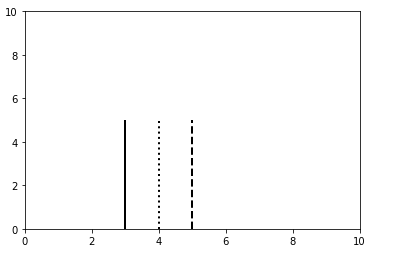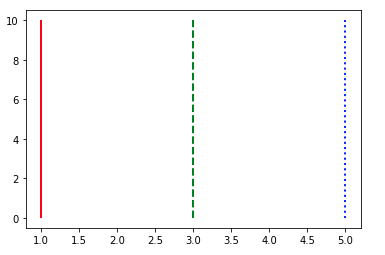# Matplotlib.pyplot.vlines() in Python

• Last Updated : 18 Nov, 2021

Matplotlib is a plotting library for creating static, animated, and interactive visualizations in Python.

## Matplotlib.pyplot.vlines()

matplotlib.pyplot.vlines() is a function used in the plotting of a dataset. In matplotlib.pyplot.vlines(), vlines is the abbreviation for vertical lines.what this function does is very much clear from the expanded form, which says that function deals with the plotting of the vertical lines across the axes.

`Syntax: vlines(x, ymin, ymax, colors, linestyles)`

Example 1:

## Python3

 `import` `numpy as np``import` `matplotlib.pyplot as plt` `plt.vlines(``4``, ``0``, ``5``, linestyles ``=``"dotted"``, colors ``=``"k"``)``plt.vlines(``3``, ``0``, ``5``, linestyles ``=``"solid"``, colors ``=``"k"``)``plt.vlines(``5``, ``0``, ``5``, linestyles ``=``"dashed"``, colors ``=``"k"``)` `plt.xlim(``0``, ``10``)``plt.ylim(``0``, ``10``)` `plt.show()`

Output :In the above example, the first argument at the beginning of the vlines() function shows the axis-point at the vertical line is to be generated, the next argument refers to the lower limit of the total length of the vertical line, whereas the third argument refers to the upper limit of the total length of the vertical line. After these three basic arguments, the next argument we can use is linestyles which decides the type of line to be plotted (eg.dotted, solid, dashed, dashdot, etc.), the last argument used is colors which is optional, basically, it is array-like of colors.
Example 2:

## Python3

 `import` `matplotlib.pyplot as plt` `plt.vlines((``1``, ``3``, ``5``,), ``0``, ``10``, colors ``=` `(``"r"``, ``"g"``, ``"b"``),``         ``linestyles ``=` `(``"solid"``, ``"dashed"``, ``"dotted"``))` `plt.show()`

Output:My Personal Notes arrow_drop_up